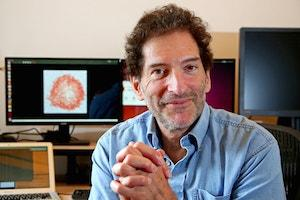Complexity Explorer Santa Fe InstituteRandom Walks

The goal of this tutorial is to outline some elementary, but beautiful aspects of random walks.  Random walks are ubiquitous in nature.  They naturally arise in describing the motion of microscopic particles, such as bacteria or pollen grains, whose motion is governed by being buffeted by collisions with the molecules in a surrounding fluid.  Random walks also control many type of fluctuation phenomena that arise in finance.

The tutorial begins by presenting examples of random walks in nature and summarizing important classes of random walks.  We'll then give a quantitative discussion of basic properties of random walks.  We'll show that the root mean-square displacement of a random walk grows as the square-root of the elapsed time.  Next, we will determine the underlying probability distribution of a random walk.  In the long-time limit, this distribution is independent of almost all microscopic details of the random-walk motion.  This universality is embodied by the central-limit theorem.  In addition to presenting this theorem, we'll also discuss the anomalous features that arise when the very mild conditions that underlie the central-limit theorem are not satisfied.  Finally, we will show how to recover the diffusion equation as the continuum limit of the evolution equation for the probability distribution of a random walk.

We will then present some basic first-passage properties of random walks, which address the following simple question: does a random walk reach a specified point for the first time?  We will determine the first-passage properties in a finite interval; specifically, how long does it take for a random walk to leave an interval of length L, and what is the probability to leave either end of the interval as a function of the starting location.  Finally, we'll discus the application of first-passage ideas to reaction-rate theory, which defines how quickly diffusion-controlled chemical reactions can occur.

Note that Complexity Explorer tutorials are meant to introduce students to various important techniques and to provide illustrations of their application in complex systems.  A given tutorial is not meant to offer complete coverage of its topic or substitute for an entire course on that topic.

This tutorial is designed for more advanced math students.  Math prerequisites for this course are an understanding of calculus, basic probability, and Fourier transforms.

Sid Redner is a Resident Faculty Member at the Santa Fe Institute.Sid Redner received an A.B. in physics from the University of California, Berkeley in 1972 and a Ph.D. in Physics from MIT in 1977.  After a postdoctoral year at the University of Toronto, Sid joined the physics faculty at Boston University in 1978.  During his 36 years at BU, he served as Acting Chair during two separate terms and also served as Departmental Chair.  Sid has been a Visiting Scientist at Schlumberger-Doll Research in the mid 80's, the Ulam Scholar at LANL in 2004, and a sabbatical visitor at Université Paul Sabatier in Toulouse France and at Université Pierre-et-Marie-Curie in Paris.

Sid has published more than 250 articles in major peer-reviewed journals, as well as two books: the monograph A Guide to First-Passage Processes (Cambridge Univ. Press, 2001) and the graduate text, jointly with P. L. Krapivsky and E. Ben-Naim, A Kinetic View of Statistical Physics (Cambridge Univ. Press, 2010).  He also a member of the Editorial Board for the Journal of Informetrics, an Associate Editor for the Journal of Statistical Physics, and a Divisional Associate Editor for Physical Review Letters.   For more on Sid, visit his website.  Read a Q&A with Sid on his Random Walks tutorial here

How to use Complexity Explorer
Enrolled students:

2,491

View Participant Map

Prerequisites:

Calculus; Basic Probability; Fourier Transforms

Like this tutorial?

4 Student Ratings

Syllabus

1. Introduction
2. Root Mean Square Displacement
3. Role of the Spatial Dimension
4. Probability Distribution and Diffusion Equation
5. Central Limit Theorem
6. First Passage Phenomena
7. Elementary Applications of First Passage Phenomena
8. Final Remarks
9. Homework